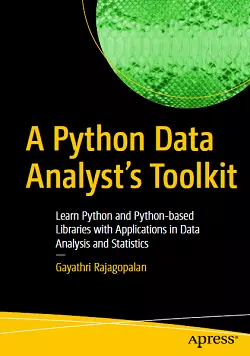# A Python Data Analyst’s Toolkit### eBook Details:

• Paperback: 419 pages
• Publisher: WOW! eBook (December 23, 2020)
• Language: English
• ISBN-10: 1484263987
• ISBN-13: 978-1484263983

### eBook Description:

Python Data Analyst’s Toolkit: Learn Python and Python-based Libraries with Applications in Data Analysis and Statistics

Explore the fundamentals of data analysis, and statistics with case studies using Python. This book will show you how to confidently write code in Python, and use various Python libraries and functions for analyzing any dataset. The code is presented in Jupyter notebooks that can further be adapted and extended.

This book is divided into three parts – programming with Python, data analysis and visualization, and statistics. You’ll start with an introduction to Python – the syntax, functions, conditional statements, data types, and different types of containers. You’ll then review more advanced concepts like regular expressions, handling of files, and solving mathematical problems with Python.

The second part of the book, will cover Python libraries used for data analysis. There will be an introductory chapter covering basic concepts and terminology, and one chapter each on NumPy (the scientific computation library), Pandas (the data wrangling library) and visualization libraries like Matplotlib and Seaborn. Case studies will be included as examples to help readers understand some real-world applications of data analysis.

What You’ll Learn

• Further your programming and analytical skills with Python
• Solve mathematical problems in calculus, and set theory and algebra with Python
• Work with various libraries in Python to structure, analyze, and visualize data
• Tackle real-life case studies using Python
• Review essential statistical concepts and use the SciPy library to solve problems in statistics

The final chapters of A Python Data Analyst‘s Toolkit book focus on statistics, elucidating important principles in statistics that are relevant to data science. These topics include probability, Bayes theorem, permutations and combinations, and hypothesis testing (ANOVA, Chi-squared test, z-test, and t-test), and how the SciPy library enables simplification of tedious calculations involved in statistics.